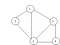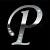# Java Files

File handling is an important part of any application.

Java has several methods for creating, reading, updating, and deleting files.

# Java File Handling

The `File` class from the `java.io` package, allows us to work with files.

To use the `File` class, create an object of the class, and specify the filename or directory name:

Example

`import java.io.File;  // Import the File classFile myObj = new File("filename.txt"); // Specify the filename`

Java Create and Write To Files

Create a File

To create a file in Java, you can use the `createNewFile()` method. This method returns a boolean value: `true` if the file was…

# Packages, Access Specifiers, Exception Handling

The package is a group of related classes and interfaces. The main objective is code reusability. Any class once after derived successfully can be accessed by importing the respective package. package can be created with the help of keyword package.

Syntax:

`package <pkg_name>class classname{  //Data Members and Methods}`

Packages are two different types

1. Predefined packages
2. User defined packages

The predefined packages are Java and Javax . The package consists of many sub packages.

Depending on the requirement of the user the respective package and the sub package will be imported into the user program using the keyword “import”.

# STACKS & QUEUES

STACK: Introduction to Stacks, Basic Stack operations, Representation of a Stack using Array, Linked list representation of stacks, operations on linked stack, stack applications: Reversing list, Factorial calculation, infix to post fix transformations, evaluating arithmetic expressions.

QUEUES: Introduction to Queues, Basic queue operations, Representation of a queue using Arrays and using Linked List, Implementation of queue operations using stack, applications of queues — circular queues, dequeues, priority queues, Multiple Queues.

A Stack is an ordered collection of data items, into which new items may be inserted and from which items may be deleted at one end called Top of the…

# Data Structures

## Definition, Classification of Data structures, Operations on data structures, Abstract data Type (ADT), Preliminaries of algorithms, Time and space complexity. Searching — Linear search, Binary search, Fibonacci search. Sorting — insertion sort, Selection sort, exchange sort(bubble sort, quick sort), distribution sort(radix sort) and merging (merge sort) algorithms.

DATA STRUCTURES : Data may be organized in many different ways. The logical or mathematical model of a particular organization of data is called a data structure.

Example: Arrays, Linked lists, Trees, Structures, Stacks, Queues, Graphs, Algebraic expressions

PERFORMANCE ANALYSIS

1. Space Complexity
2. Time Complexity

Space Complexity: The space complexity of an algorithm is the amount of memory it needs to run to completion.

Time Complexity: The time complexity of an algorithm is the amount of computer time it needs to run to completion.

Space Complexity: The space needed by the algorithms is seen to be the sum of the following…

# Sorting Algorithms

Arranging the elements in ascending order or in descending order is called Sorting. Sorting techniques are broadly categorized into two.

1. Internal Sorting and
2. External Sorting.

1. Internal Sorting : All the records that are to be sorted are in main memory.

2. External Sorting: Some sorts that cannot be performed in main memory and must be done on disk or tape. This type of sorting is known as External Sorting.

Efficiency: One of the major issues in the sorting algorithms is its efficiency. If we can efficiently sort the records then that adds value to the sorting algorithm. We usually…

# Graphs — Introduction, DFS, BFS, Prims Algorithm, Kruskal’s Algorithm and their Implementations

A graph G consists of a set of V or vertices (nodes) and a set if edges (arcs). Vertices are nothing but the nodes in the graph. Two adjacent vertices are joined by edges.

We write G = (V, E).

V is a finite non empty set of vertices.

E is a set of pairs of vertices. These pairs are called edges.

An edge e=(v, w), is a pair of vertices v and w, and is said to be incident with v and w. There are two types of Graphs i) Undirected graphs and ii) Directed graphs.

Undirected Graph:Undirected Graph

V(G)=…

# TREES- Binary Trees, Binary Search Trees, AVL Trees

A Tree is a finite set of one or more nodes such that

i) There is a specially designated node called the Root.

ii) The remaining nodes are partitioned into n > = 0 disjoint sets T1 T2 T3…. Tn. Where each of these sets is a Tree.

A node stands for the item of information plus the branches to other items.

The number of subtrees of a node is called its degree. Nodes that have degree ZERO are called Leaf nodes or Terminal nodes.

There are many applications for trees . One of the popular uses is the directory…

# Dynamic Memory Allocation

• Allocating and Freeing dynamic variables
• Drawbacks of Arrays
• Need for dynamic storage
• Linked Lists

Consider the following array declaration

`int marks;`

Such a declaration would typically be used if 100 students marks are to be stored in memory. The moment we make this declaration 200 bytes one reserved in memory. For storing 100 integers in it. However, it may so happen that when we actually run the program we might be interested in storing only 60 students marks. Even in this case 200 bytes would get reserved in memory, which would result in wastage of memory.

Another possibility is that…

# Inheritance

It is the ability to extract the properties of one class into another class.

The main objective is to provide code reusability. It reduces the code redundancy to a greater extent which saves lot of developer time.

## Types of Inheritance:

1. Single
2. Multi level
3. Hierarchical

Multiple and Hybrid inheritances can be achieved through Interfaces but not through classes.

In order to inherit one class to another class we use the keyword “extends”.

# Single Inheritance

Here only two classes are involved in which one is parent class and the other one is the child class.

Once A is inherited into B we need not create object for…

# Java OOPs Concept, Constructors, PolyMorphism, Constructor Overloading, Static Keyword

## Object Oriented Features

OOPs is a method of implementation where a co-operative collection of objects may be created, accessed and deployed.

Features:

1. Classes and Objects
2. Data Abstraction
3. Data Encapsulation
4. Polymorphism
5. Inheritance
6. Persistence
7. Extendability
8. Message Passing

## Class

A class is a description of similar kind of objects or class is a blue print for several objects. A class is a logical entity that gives description of related objects.

## Object

Object is a real world entity or An object is an instance of a class. An object is a physical entity which occupies certain space in memory.

## Data Abstraction

It is a process of exhibiting the essential details by…